# 深度学习利器：TensorFlow 实战

## 深度学习及 TensorFlow 简介

TensorFlow 开源一周年以来，已有 500+contributors，以及 11000+ 个 commits。目前采用 TensorFlow 平台，在生产环境下进行深度学习的公司有 ARM、Google、UBER、DeepMind、京东等公司。目前谷歌已把 TensorFlow 应用到很多内部项目，如谷歌语音识别，GMail，谷歌图片搜索等。TensorFlow 主要特性有：

## TensorFlow 变量

TensorFlow 中的变量在使用前需要被初始化，在模型训练中或训练完成后可以保存或恢复这些变量值。下面介绍如何创建变量，初始化变量，保存变量，恢复变量以及共享变量。

#创建模型的权重及偏置  weights = tf.Variable(tf.random_normal([784, 200], stddev=0.35), name="weights") biases = tf.Variable(tf.zeros(), name="biases")   #指定变量所在设备为 CPU:0 with tf.device("/cpu:0"):   v = tf.Variable(...)   #初始化模型变量  init_op = tf.global_variables_initializer() sess=tf.Session() sess.run(init_op)   #保存模型变量，由三个文件组成 model.data，model.index，model.meta saver = tf.train.Saver() saver.restore(sess, "/tmp/model")   #恢复模型变量  saver.restore(sess, "/tmp/model")

#定义卷积神经网络运算规则，其中 weights 和 biases 为共享变量  def conv_relu(input, kernel_shape, bias_shape):     # 创建变量"weights".     weights = tf.get_variable("weights", kernel_shape, initializer=tf.random_normal_initializer())     # 创建变量 "biases".     biases = tf.get_variable("biases", bias_shape, initializer=tf.constant_initializer(0.0))     conv = tf.nn.conv2d(input, weights, strides=[1, 1, 1, 1], padding='SAME')     return tf.nn.relu(conv + biases)   #定义卷积层，conv1 和 conv2 为变量命名空间  with tf.variable_scope("conv1"):     # 创建变量 "conv1/weights", "conv1/biases".     relu1 = conv_relu(input_images, [5, 5, 32, 32], ) with tf.variable_scope("conv2"):     # 创建变量 "conv2/weights", "conv2/biases".     relu1 = conv_relu(relu1, [5, 5, 32, 32], )

## TensorFlow 应用架构

TensorFlow 的应用架构主要包括模型构建，模型训练，及模型评估三个方面。模型构建主要指构建深度学习神经网络，模型训练主要指在 TensorFlow 会话中对训练数据执行神经网络运算，模型评估主要指根据测试数据评估模型精确度。如下图所示：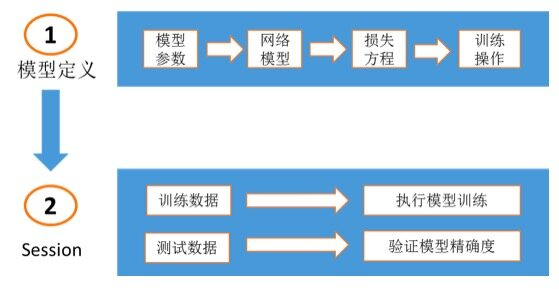#两个隐藏层，一个 logits 输出层  hidden1 = tf.nn.relu(tf.matmul(images, weights) + biases) hidden2 = tf.nn.relu(tf.matmul(hidden1, weights) + biases) logits = tf.matmul(hidden2, weights) + biases   #损失方程，采用 softmax 交叉熵算法  cross_entropy = tf.nn.sparse_softmax_cross_entropy_with_logits( logits, labels, name='xentropy') loss = tf.reduce_mean(cross_entropy, name='xentropy_mean')   #选定优化算法及定义训练操作  optimizer = tf.train.GradientDescentOptimizer(learning_rate) global_step = tf.Variable(0, name='global_step', trainable=False) train_op = optimizer.minimize(loss, global_step=global_step)   模型训练及模型验证示例如下： #加载训练数据，并执行网络训练  for step in xrange(FLAGS.max_steps):     feed_dict = fill_feed_dict(data_sets.train, images_placeholder, labels_placeholder)     _, loss_value = sess.run([train_op, loss], feed_dict=feed_dict)   #加载测试数据，计算模型精确度  for step in xrange(steps_per_epoch):     feed_dict = fill_feed_dict(data_set, images_placeholder, labels_placeholder)     true_count += sess.run(eval_correct, feed_dict=feed_dict)

## TensorFlow 可视化技术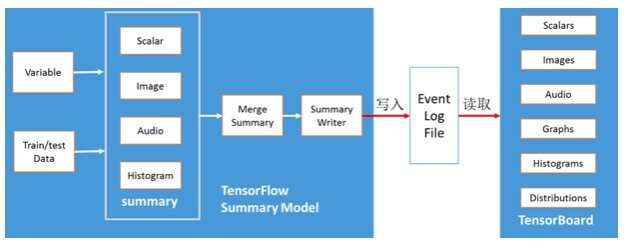TensorFlow 可视化技术主要分为两部分：TensorFlow 摘要模型及 TensorBoard 可视化组件。在摘要模型中，需要把模型变量或样本数据转换为 TensorFlow summary 操作，然后合并 summary 操作，最后通过 Summary Writer 操作写入 TensorFlow 的事件日志。TensorBoard 通过读取事件日志，进行相关摘要信息的可视化展示，主要包括：Scalar 图、图片数据可视化、声音数据展示、图模型可视化，以及变量数据的直方图和概率分布图。TensorFlow 可视化技术的关键流程如下所示：

#定义变量及训练数据的摘要操作  tf.summary.scalar('max', tf.reduce_max(var)) tf.summary.histogram('histogram', var) tf.summary.image('input', image_shaped_input, 10)   #定义合并变量操作，一次性生成所有摘要数据  merged = tf.summary.merge_all()   #定义写入摘要数据到事件日志的操作  train_writer = tf.train.SummaryWriter(FLAGS.log_dir + '/train', sess.graph) test_writer = tf.train.SummaryWriter(FLAGS.log_dir + '/test')   #执行训练操作，并把摘要信息写入到事件日志  summary, _ = sess.run([merged, train_step], feed_dict=feed_dict(True)) train_writer.add_summary(summary, i)     #下载示例 code，并执行模型训练    python mnist_with_summaries.py   #启动 TensorBoard，TensorBoard 的 UI 地址为 http://ip_address:6006 tensorboard --logdir=/path/to/log-directory

TensorBoard Scalar 图如下所示，其中横坐标表示模型训练的迭代次数，纵坐标表示该标量值，例如模型精确度，熵值等。TensorBoard 支持这些统计值的下载。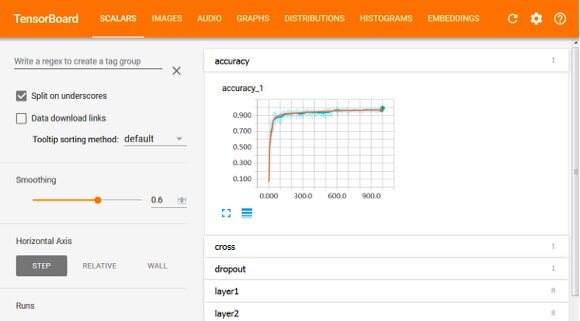TensorFlow Image 摘要信息如下图所示，该示例中显示了测试数据和训练数据中的手写数字图片。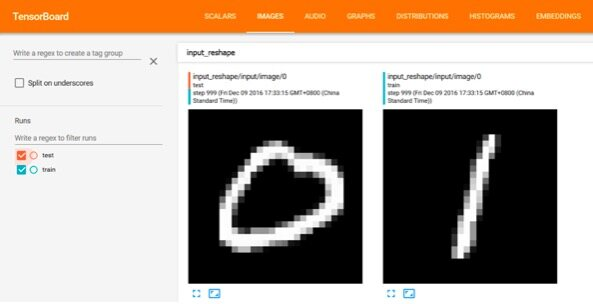TensorFlow 图模型如下图所示，可清晰地展示模型的训练流程，其中的每个方框表示变量所在的命名空间。包含的命名空间有 input（输入数据），input_reshape（矩阵变换，用于图形化手写数字）, layer1（隐含层 1）, layer2（隐含层 2）, dropout（丢弃一些神经元，防止过拟合）, accuracy（模型精确度）, cross_entropy（目标函数值，交叉熵）, train（训练模型）。例如，input 命名空间操作后的 tensor 数据会传递给 input_reshape，train，accuracy，layer1，cross_entropy 命名空间中的操作。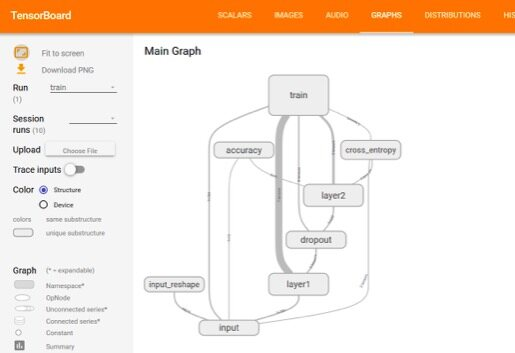TensorFlow 变量的概率分布如下图所示，其中横坐标为迭代次数，纵坐标为变量取值范围。图表中的线表示概率百分比，从高到底为 [maximum, 93%, 84%, 69%, 50%, 31%, 16%, 7%, minimum]。例如，图表中从高到底的第二条线为 93%，对应该迭代下有 93% 的变量权重值小于该线对应的目标值。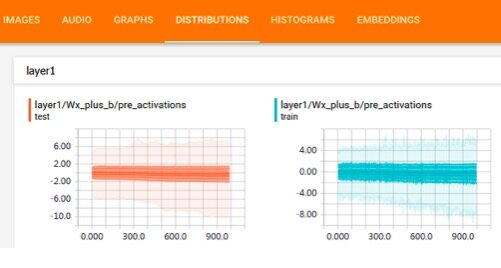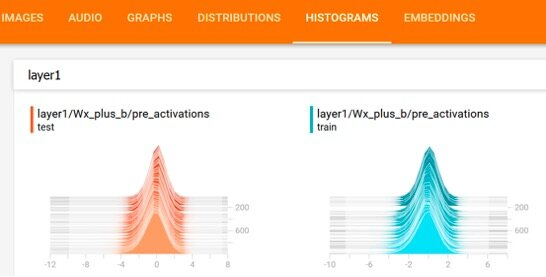## TensorFlow GPU 使用

GPU 设备已经广泛地应用于图像分类，语音识别，自然语言处理，机器翻译等深度学习领域，并实现了开创性的性能改进。与单纯使用 CPU 相比，GPU 具有数以千计的计算核心，可实现 10-100 倍的性能提升。TensorFlow 支持 GPU 运算的版本为 tensorflow-gpu，并且需要先安装相关软件：GPU 运算平台 CUDA 和用于深度神经网络运算的 GPU 加速库 CuDNN。在 TensorFlow 中，CPU 或 GPU 的表示方式如下所示：

"/cpu:0"：表示机器中第一个 CPU。

"/gpu:0"：表示机器中第一个 GPU 卡。

"/gpu:1"：表示机器中第二个 GPU 卡。

TensorFlow 中所有操作都有 CPU 和 GPU 运算的实现，默认情况下 GPU 运算的优先级比 CPU 高。如果 TensorFlow 操作没有指定在哪个设备上进行运算，默认会优选采用 GPU 进行运算。下面介绍如何在 TensorFlow 使用 GPU：

# 定义使用 gpu0 执行 a*b 的矩阵运算，其中 a，b，c 都在 gpu0 上执行  with tf.device('/gpu:0'):   a = tf.constant([1.0, 2.0, 3.0, 4.0, 5.0, 6.0], shape=[2, 3], name='a')   b = tf.constant([1.0, 2.0, 3.0, 4.0, 5.0, 6.0], shape=[3, 2], name='b')   c = tf.matmul(a, b)   # 通过 log_device_placement 指定在日志中输出变量和操作所在的设备  sess = tf.Session(config=tf.ConfigProto(log_device_placement=True)) print sess.run(c)

#设备的 Device Mapping /job:localhost/replica:0/task:0/gpu:0 -> device: 0, name: Tesla K20c, pci bus id: 0000:81:00.0   #变量操作所在设备位置  a: (Const): /job:localhost/replica:0/task:0/gpu:0 b: (Const): /job:localhost/replica:0/task:0/gpu:0 (MatMul)/job:localhost/replica:0/task:0/gpu:0

#定义 TensorFlow 配置  config = tf.ConfigProto() #配置 GPU 内存分配方式  #config.gpu_options.allow_growth = True #config.gpu_options.per_process_gpu_memory_fraction = 0.4 session = tf.Session(config=config, ...)

## TensorFlow 与 HDFS 集成使用

HDFS 是一个高度容错性的分布式系统，能提供高吞吐量的数据访问，非常适合大规模数据集上的应用。TensorFlow 与 HDFS 集成示例如下：

#配置 JAVA 和 HADOOP 环境变量  source $HADOOP_HOME/libexec/hadoop-config.sh export LD_LIBRARY_PATH=$LD_LIBRARY_PATH:$JAVA_HOME/jre/lib/amd64/server #执行 TensorFlow 运行模型 CLASSPATH=$(\$HADOOP_HDFS_HOME/bin/hadoop classpath --glob) python tensorflow_model.py   #在 TensorFlow 模型中定义文件的读取队列  filename_queue = tf.train.string_input_producer(["hdfs://namenode:8020/path/to/file1.csv", "hdfs://namenode:8020/path/to/file2.csv"])   #从文件中读取一行数据，value 为所对应的行数据  reader = tf.TextLineReader() key, value = reader.read(filename_queue)   # 把读取到的 value 值解码成特征向量，record_defaults 定义解码格式及对应的数据类型  record_defaults = [, , , , ] col1, col2, col3, col4, col5 = tf.decode_csv(value, record_defaults=record_defaults) features = tf.pack([col1, col2, col3, col4])   with tf.Session() as sess:   # 定义同步对象，并启动相应线程把 HDFS 文件名插入到队列    coord = tf.train.Coordinator()   threads = tf.train.start_queue_runners(coord=coord)     for i in range(1200):     # 从文件队列中读取一行数据      example, label = sess.run([features, col5])      #请求停止队列的相关线程（包括进队及出队线程）   coord.request_stop()   #等待队列中相关线程结束（包括进队及出队线程）   coord.join(threads)

## 作者简介